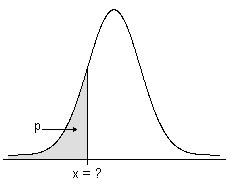概率分布图的方法和公式

累积分布函数

• 对于连续分布，CDF 给出概率密度函数下的区域，最多至您指定的 x 值。
• 对于离散分布，CDF 给出您指定的 x 值的累积概率。

逆累积概率ICDF 是与概率密度函数下的区域关联的值。ICDF 是累积分布函数 (CDF) 的反函数，累积分布函数是与某值相关联的区域。

• 当概率密度函数 (PDF) 对整个实数行表示为正值（例如，正态 PDF）时，不会为 p = 0 或 p = 1 定义 ICDF。
• 当 PDF 对于大于某值的所有值表示为正值（例如卡方 PDF）时，将为 p =0 定义 ICDF，但不为 p =1 进行定义。
• 当 PDF 仅在某区间上才为正值时（例如，均匀 PDF），将为 p = 0 和 p = 1 定义 ICDF。
• 在未定义 ICDF 时，Minitab 会为结果返回一个缺失值 (*)。

概率密度函数

• 对于连续分布，X 在区间 (a, b) 中具有值的概率是其在区间 (a, b) 中的 PDF 下的区域。
• 对于离散分布，X 在区间 (a, b) 中具有值的概率是 (a, b) 中 X 的可能离散值的 PDF（也称为概率密度函数）之和。

Beta 分布

Beta 分布常用于表示具有自然上限和下限的过程。

α 形状参数 1
β 形状参数 2
Γ Gamma 函数
a 下限
b 上限

a = 0 时，b = 1，

PDF 为：

n 试验数
x 事件数
p 事件概率

Cauchy 分布

Cauchy 分布沿零对称，但其尾部接近零的速度要比正态分布慢。

a 位置参数
b 尺度参数
π Pi (~3.142)

卡方分布

n 个独立 X2 变量（其中 X 具有标准正态分布）的总和具有卡方分布（自由度为 n）。卡方分布的形状取决于自由度的数量。

ν 自由度
ΓGamma 函数
e 自然对数的基数

−1 0.2
0 0.5
1 0.3

θ 尺度参数
λ 阈值参数
exp 自然对数的基数

F 分布

F 分布也称为方差比值分布，具有两种类型的自由度：分子自由度和分母自由度。它是两个独立的带有卡方分布的随机变量（每个变量被其自由度所除）的比值的分布。

Γ Gamma 函数
u 分子自由度
v 分母自由度

Gamma 分布

Gamma 分布通常用于对正向偏斜的数据建模。

表示法

a 形状参数（当 a = 1 时，Gamma PDF 与指数分布 PDF 相同）
b 尺度参数
θ 阈值参数
Γ Gamma 函数
e 自然对数的基数

几何分布

表示法

X 生成一个事件所需执行的试验数， Y + 1
Y 在第一个事件之前发生的非事件数
p 事件在每个试验中的发生概率

NN1 + N2 = 总体大小
N1 总体中的事件数
N2 总体中的非事件数
n 样本数量
x 样本中的事件数

a 位置参数
b 尺度参数
e 自然对数的基数

最大极值分布

表示法

σ 尺度参数
μ 位置参数
γ Euler 常数 (~0.57722)

μ 位置参数
σ 尺度参数

μ 位置参数
σ 尺度参数
λ 阈值参数
Γ Gamma 函数
exp 自然对数的基数

μ 位置参数
σ 尺度参数
λ 阈值参数
π Pi (~3.142)

负二项分布

公式X Y + r
r 事件数
p 事件的概率

exp自然对数的基数
π Pi (~3.142)

Poisson 分布

Poisson 分布是可对基于恒定发生率的事件数量建模的离散分布。当独立试验数量大并且成功概率小时，Poisson 分布可用作二项式的近似。

e 自然对数的基数

最小极值分布

表示法

ξ 位置参数
θ 尺度参数
e 自然对数的基数
v Euler 常数 (~0.57722)

t 分布

• 创建正态分布中的总体均值的置信区间（当方差未知时）。
• 确定正态总体中的两个样本均值（具有未知但相等的方差）是否显著不同。
• 检验回归系数的显著性。

Γ Gamma 函数
v 自由度
π Pi (~3.142)

三角分布

表示法

a 下端点
b 上端点
c 模式（PDF 尖峰所在的位置）

a 下端点
b 上端点

Weibull 分布

Weibull 分布可对产品失效时间进行建模。

表示法

α 尺度参数
β 形状参数，当 β = 1 时，Weibull PDF 与指数分布 PDF 相同
λ 阈值参数
Γ Gamma 函数
exp 自然对数的基数